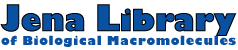[JenaLib Home]     [Helix analysis Home]     [JenaLib Entry]     [Sequence, Chains, Units]     [Helix analysis Entry]     [Bending Classification]

# Bending of the helical axis

 Title THE PHAGE 434 CRO/OR1 COMPLEX AT 2.5 ANGSTROMS RESOLUTION PDB code 3CRO   (PDB summary) NDB code PDR001 (NDB atlas) Duplex length 19 base pairs Protein CRO protein, Transcription factor, DNA binding domain: Prokaryotic helix-turn-helix

Table 1.   Bending parameters determined with the program ARC_FIT.
The curvilinear helical axis is calculated with CURVES, and bending of this axis is analysed with ARC_FIT. This program allows to fit a straight line, a plane, an arc, a kinked line, and a double kinked line to the helical axis. Good fits are indicated by low values of sigma2. An arc is fitted if there are at least 7 bp. A kinked line requires at least 8 bp, and the double kinked line requires at least 13 bp. The geometrical parameters are given for all models fitted, but they characterize the shape of the helical axis only if the fit is good. See Help for further explanations.

 Model sigma2 / Å2 Geometrical parameters Plane 0.099 Straight line (x-axis) 4.059 Arc 0.271 Radius of curvature:  r = 83 Å Kinked line 0.787 Kink angle:  alpha = 22° Lengths of straight segments:  l1 = 9 bp,  l2 = 10 bp Double kinked line 0.145 Kink angles:  alpha1 = 18°,  alpha2 = 18°   Twist angle:  omega = -20°   Lengths of straight segments:  l1 = 6 bp,  l2 = 8 bp,  l3 = 5 bp